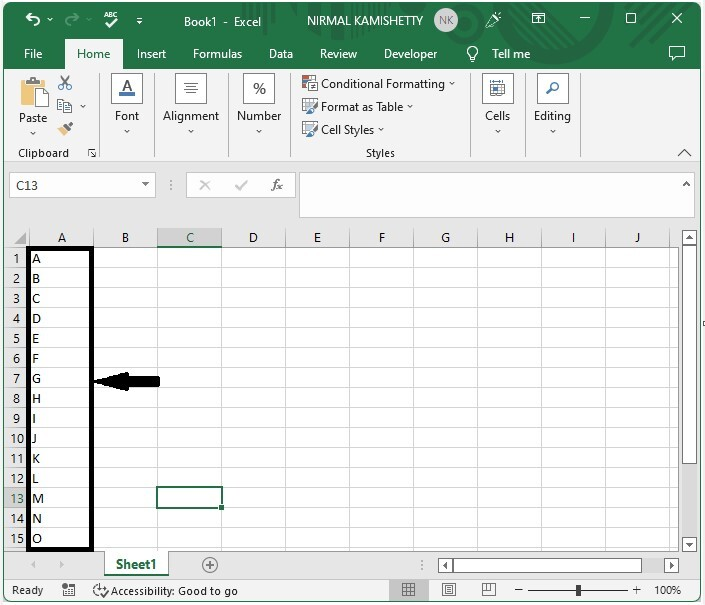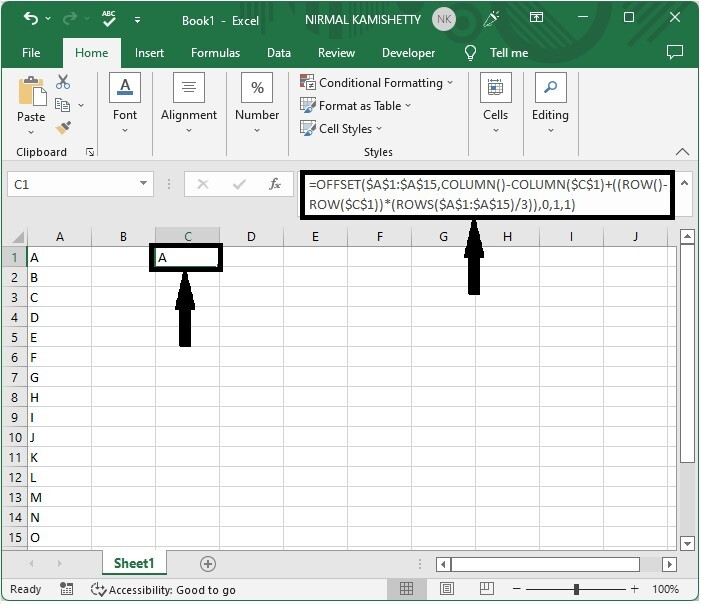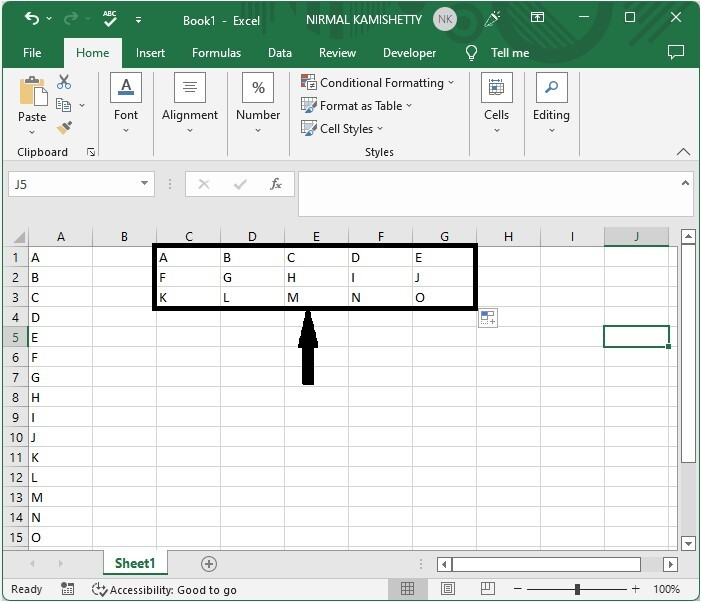# How to Convert Vector / Single Row or Column to Matrix in Excel?

A matrix is rectangular array with n rows and m columns. Sometimes in excel when we have a data in single column or single row you would want to convert them into a matrix. If you try to solve this manually, then it can be a time-consuming process, as we need to copy each row manually and paste them. So let us see a faster process.

We can solve this using the formulas supported by Excel. The only condition for the matrix is that the all the values must be filled in whole "m*n" values. This tutorial will help you to understand how we can convert vector or single column to matrix in Excel.

## Converting a Single Column to Matrix in Excel

Here we will use the formula to get a single value and then fill all the values using the auto-fill handler. Let's look at a simple procedure for converting a single column to a matrix in Excel.

### Step 1

Let us consider an Excel sheet where the data is a list of elements similar to the below image.Then, in our case, cell C1, click on an empty cell and enter the formula as follows −

=OFFSET($A$1:$A$15,COLUMN()-COLUMN($C$1)+((ROW()-ROW($C$1))*(ROWS($A$1:$A$15)/3)),0,1,1)


and click Enter to get our first result. In the formula, A1:A15 is the range of our values, C1 is the address of our current cell, and 3 is the number of rows you want to create in the matrix.

Empty cell > Formula > Enter### Step 2

Now drag in both right and down directions using the auto-fill handle to create a matrix similar to the below image.If we want to create a matrix based on a row, we can use the same process, but the only change is that the formula will be changed to

=OFFSET($A$1:$J$1,0,COLUMN()-COLUMN($C$2)+(ROW()-ROW($C$2))*(COLUMNS($A$1:$J$1)/5),1,1).


## Conclusion

In this tutorial, we used a simple example to demonstrate how you can convert a single column to a matrix in Excel.

Updated on: 07-Mar-2023

1K+ Views